## Everything You Wanted to Know about the Kernel Trick

### (But Were Too Afraid to Ask)

Eric Kim (eric.kim.cs@gmail.com, http://www.eric-kim.net)

## Abstract

The goal of this writeup is to provide a high-level introduction to the "Kernel Trick" commonly used in classification algorithms such as Support Vector Machines (SVM) and Logistic Regression. My target audience are those who have had some basic experience with machine learning, yet are looking for an alternative introduction to kernel methods.

We first examine an example that motivates the need for kernel methods. After an explanation about the "Kernel Trick", we finally apply kernels to improve classification results.

The following code examples are in Python, and make heavy use of the sklearn, numpy, and scipy libraries. I have made the code used in this writeup available - head to the bottom of the article for links to the source files.

## So, What is a Kernel Anyway?

A Kernel Function $K(\vec{v},\vec{w})$ is a function $K: \mathbb{R}^N \times \mathbb{R}^N \rightarrow \mathbb{R}$ [notation] that obeys certain mathematical properties. I won't go into these properties right now, but for now think of a kernel as a function that computes a dot product between $\vec{v}$, $\vec{w}$ (e.g. a measure of 'similarity' between $\vec{v}, \vec{w}$).

Unimpressed? Me too! Don't worry, we'll examine its significance in greater detail.

## Linear SVM, Binary Classification

Suppose that we have a two-class dataset $D$, and we wish to train a classifier $C$ to predict the class labels of future data points. This is known as a "binary classification" problem, and can be cast as "Yes"/"No" questions such as:

• Medicine
• Given a patient's vital data, does the patient have a cold?
• Computer Vision
• Does this image contain a person?
A popular off-the-shelf classifier is the Support Vector Machine (SVM), so we will use this as our classification algorithm.

"Binary vs. Multiclass Classification"

In most introductory courses to Machine Learning, binary classifiers are often the focus due to their simpler presentation. However, many problems inherently have more than two possible outcomes. For instance, you may want to train a face verification system that can detect the identity of a photograph from a pool of $N$ people (where $N > 2$). This sort of problem is known as a "multiclass" classification problem.

Most mathematical definitions of classifiers are originally posed as binary classifiers, including the SVM. Thus, there are two main approaches to the multiclass problem:

1. Directly add a multiclass extension to a binary classifier.
• Pros: Provides a principled way of solving the multiclass problem.
• Cons: Multiclass extensions tend to be much more complicated than the original binary formulation. This may lead to significantly longer training and test procedures. Even worse, experimental results may not be that much better than ad-hoc methods such as (2) .
2. Combine multiple binary classifiers to create a 'mega' multi-class classifier. Two popular implementations of this idea are the "One-versus-One" (OVO) and "One-versus-All" (OVA) schemes.
• Pros: Simple idea, easy to implement, can be much faster than multiclass extensions (1). Some people suggest that empirical results tend to be on par with (1) .
• Cons: Ultimately is an ad-hoc method for solving the multiclass problem - there may exist datasets for which OVO/OVA will perform poorly on, but general multiclass classifiers (1) perform well on.
Refer to  for an excellent survey of multiclass techniques. It is interesting to note that  recommends to use OVO/OVA rather than more-complicated generalized multiclass classifiers. Their justification is: OVO/OVA tends to produce similar results to (1) with significantly less computation time.

Recall that a Linear SVM finds a hyperplane $\vec{w}$ that best-separates the data points in the training set by class label. $\vec{w}$ is called the decision boundary, and cuts the space into two halves: one half for class '0', and the other half for class '1'. To classify a point $x_i \in X$ (where $X$ is the dataset), we simply see which 'side' of $\vec{w}$ that $x_i$ lies. Note that this description only applies to binary classification problems - if your dataset has more than two classes, there are other SVM approaches (such as one-versus-all, one-versus-one, etc.).

As a quick example, here's a synthetic dataset that is designed to be linearly separable: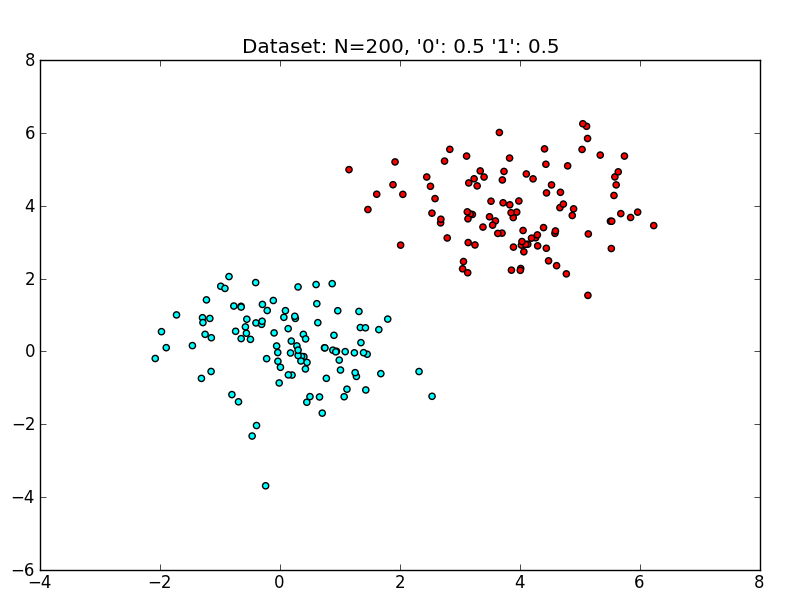Figure 1: A two-class, linearly separable dataset.

Training and evaluating a linear SVM on this dataset yields the following decision boundary (Figure 2). Because the data is easily linearly separable, the SVM is able to find a margin that perfectly separates the training data, which also generalizes very well to the test set. The hyperplane $\vec{w}$ (a line in $\mathbb{R}^2$) separates the space into two halves: points that live in the brownish region are classified as class '1', whereas points that live in the blueish region are classified as class '0'.Figure 2: The Decision Boundary of a Linear SVM on a linearly-separable dataset. The solid line is the boundary. The SVM is trained on 75% of the dataset, and evaluated on the remaining 25%. Circled data points are from the test set.

Unfortunately, in practice we will not always encounter such well-behaved datasets. Let's take a look at a dataset that is not linearly separable.

### A Linearly Nonseparable Dataset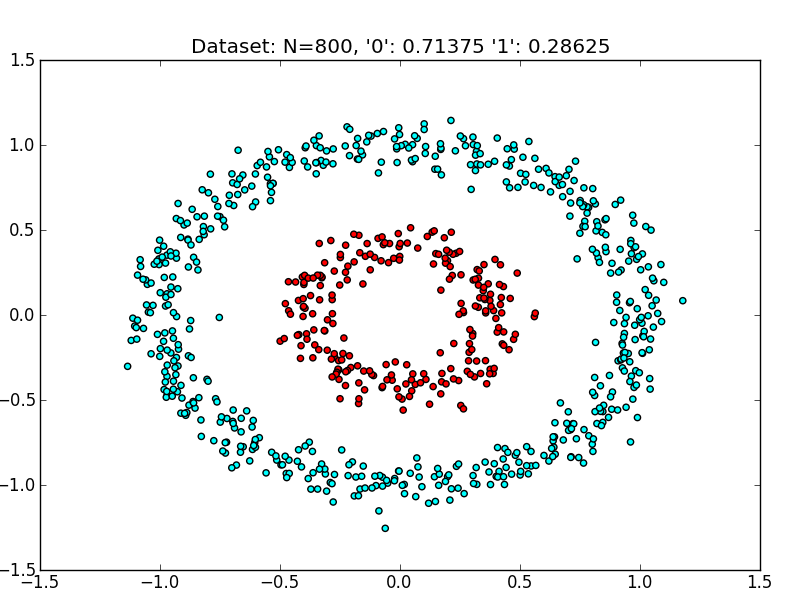Figure 3: A two-class dataset that is not linearly separable. The outer ring (cyan) is class '0', while the inner ring (red) is class '1'.

Consider the dataset in Figure 3. No line in $\mathbb{R}^2$ can reasonably separate the two classes - thus, we expect that a linear SVM will perform poorly on this dataset. As a sanity check, let's train a linear SVM on this dataset, and see just how poorly it performs. As a baseline, we will compare against a random classifier, which randomly chooses a class label ('0', '1') for each test point with uniform probability:

==== Evaluating Random Classifier
== Accuracy: 0.45
precision    recall  f1-score   support

0       0.74      0.42      0.53       151
1       0.23      0.55      0.33        49

avg / total       0.62      0.45      0.48       200

==== Finished Random Classifier (0.000 s)

==== Evaluating SVM (kernel='linear'), 2-fold cross validation
Parameters to be chosen through cross validation:
C: [1.0, 10.0, 100.0, 1000.0, 10000.0]
== Best Params: {'kernel': 'linear', 'C': 1.0}
== Best Score: 0.476666666667
== Accuracy: 0.445
precision    recall  f1-score   support

0       0.78      0.37      0.50       151
1       0.26      0.67      0.37        49

avg / total       0.65      0.45      0.47       200

==== Finished Linear SVM (1.290 s)


As we can see, the RandomClassifier and Linear SVM both perform poorly. It's always a bummer when our classifier is as bad as random guessing! To get a geometric sense of the SVM's failure to cope with this dataset, see Figure 4 for the decision boundary $\vec{w}$.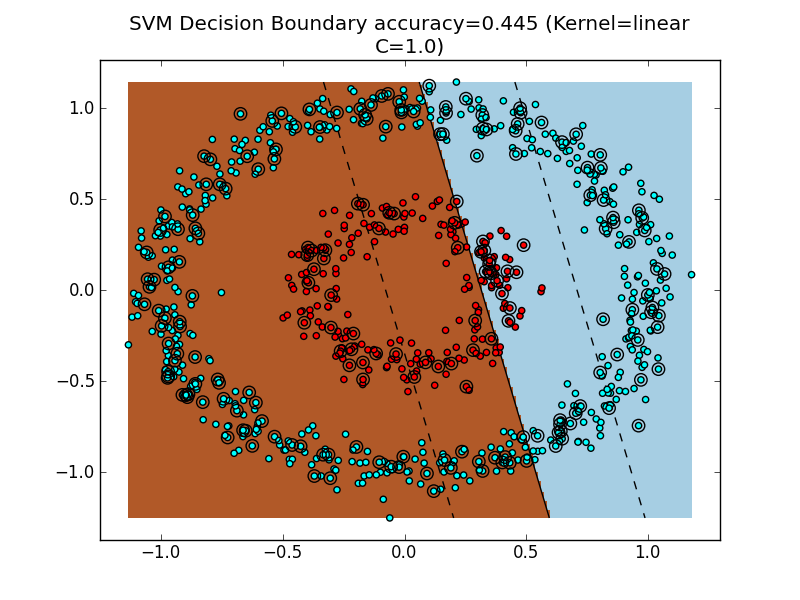Figure 4: The decision boundary of a linear SVM classifier. Because the dataset is not linearly separable, the resulting decision boundary performs and generalizes extremely poorly. Like in Figure 2, we train the SVM on 75% of the dataset, and test on the remaining 25%.

Unsurprisingly, the decision boundary fails to coherently separate the dataset, resulting in poor performance.

## Dealing with Nonseparable Data

Obviously, we would like to handle linearly nonseparable data - otherwise, SVMs wouldn't be very useful. In the SVM example, the primary obstacle is the constraint that the decision boundary be linear in the original feature space (here, $\mathbb{R}^2$). However, this is not always the correct decision boundary to find. For instance, in Figure 4, a better decision boundary would be a circular decision boundary that separates the outer cyan ring from the inner red ring.

Could we generalize the SVM formulation to explicitly discover decision boundaries with arbitrary shape? As it turns out, if you get into the nitty-gritty mathematical details of the SVM, the derivations assume that the decision boundary is a separating hyperplane $\vec{w}$. I imagine there is a way to recast the SVM optimization problem such that a more-general decision surface can be found, but I'd bet that the resulting optimization would carry a significant computational burden when compared to the linear SVM formulation.

So, it appears that we are stuck with an SVM that, for an N-dimensional dataset, finds an (N-1)-dimensional separating hyperplane. What if we could play with N...?

### Idea: Separable in higher-dimension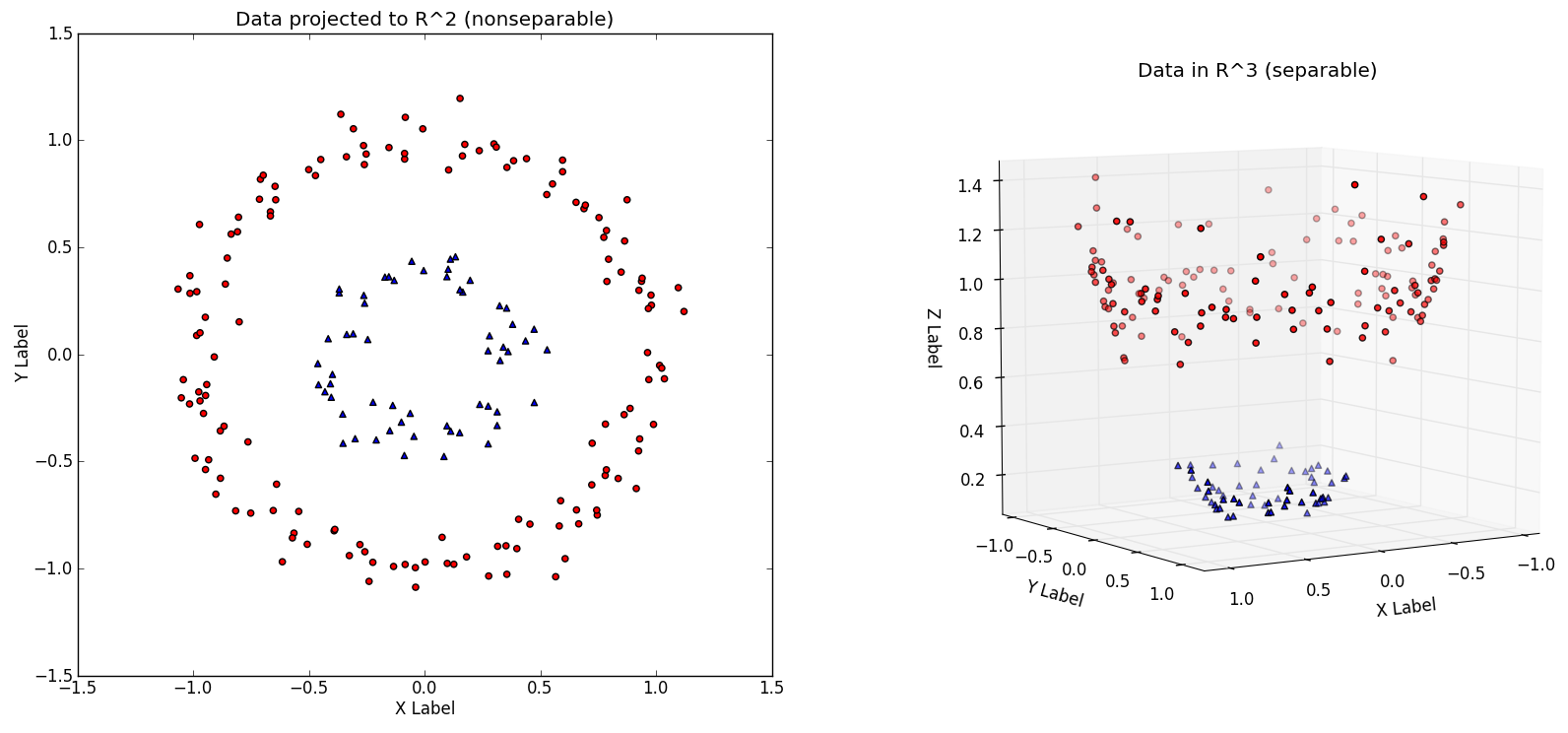Figure 5: (Left) A dataset in $\mathbb{R}^2$, not linearly separable. (Right) The same dataset transformed by the transformation: $[x_1, x_2] = [x_1, x_2, {x_1}^2 + {x_2}^2]$ .

Let's think outside the box for a moment. Consider the linearly nonseparable dataset in Figure 5 (left), with its two concentric rings. Imagine that this dataset is merely a 2-D version of the 'true' dataset that lives in $\mathbb{R}^3$, Figure 5 (right). The $\mathbb{R}^3$ dataset is easily linearly separable by a hyperplane. Thus, provided that we work in this $\mathbb{R}^3$ space, we can train a linear SVM classifier that successfully finds a good decision boundary.

However, we are given the dataset in $\mathbb{R}^2$. The challenge is to find a transformation $T: \mathbb{R}^2$ -> $\mathbb{R}^3$, such that the transformed dataset is linearly separable in $\mathbb{R}^3$. In Figure 5, the $T$ used is $T(~[x_1, x_2]~) = [x_1, x_2, {x_1}^2 + {x_2}^2]$, which after applied to every point in Figure 5 (left) yields the linearly separable dataset Figure 5 (right).

Note: It is convention to use the Greek letter 'phi' $\phi$ for this transformation $T$, so I'll use $\phi$ from here on.

Assuming we have such a transformation $\phi$, the new classification pipeline is as follows. First transform the training set $X$ to $X'$ with $\phi$. Train a linear SVM on $X'$ to get classifier $f_{svm}$. At test time, a new example $\vec{x}$ will first be transformed to $\vec{x'} = \phi(\vec{x})$. The output class label is then determined by: $f_{svm}(\vec{x'})$.

Observation: This is exactly the same as the train/test procedure for regular linear SVMs, but with an added data transformation via $\phi$.

In Figure 6, note that the hyperplane learned in $\mathbb{R}^3$ is nonlinear when projected back to $\mathbb{R}^2$. Thus, we have improved the expressiveness of the Linear SVM classifier by working in a higher-dimensional space.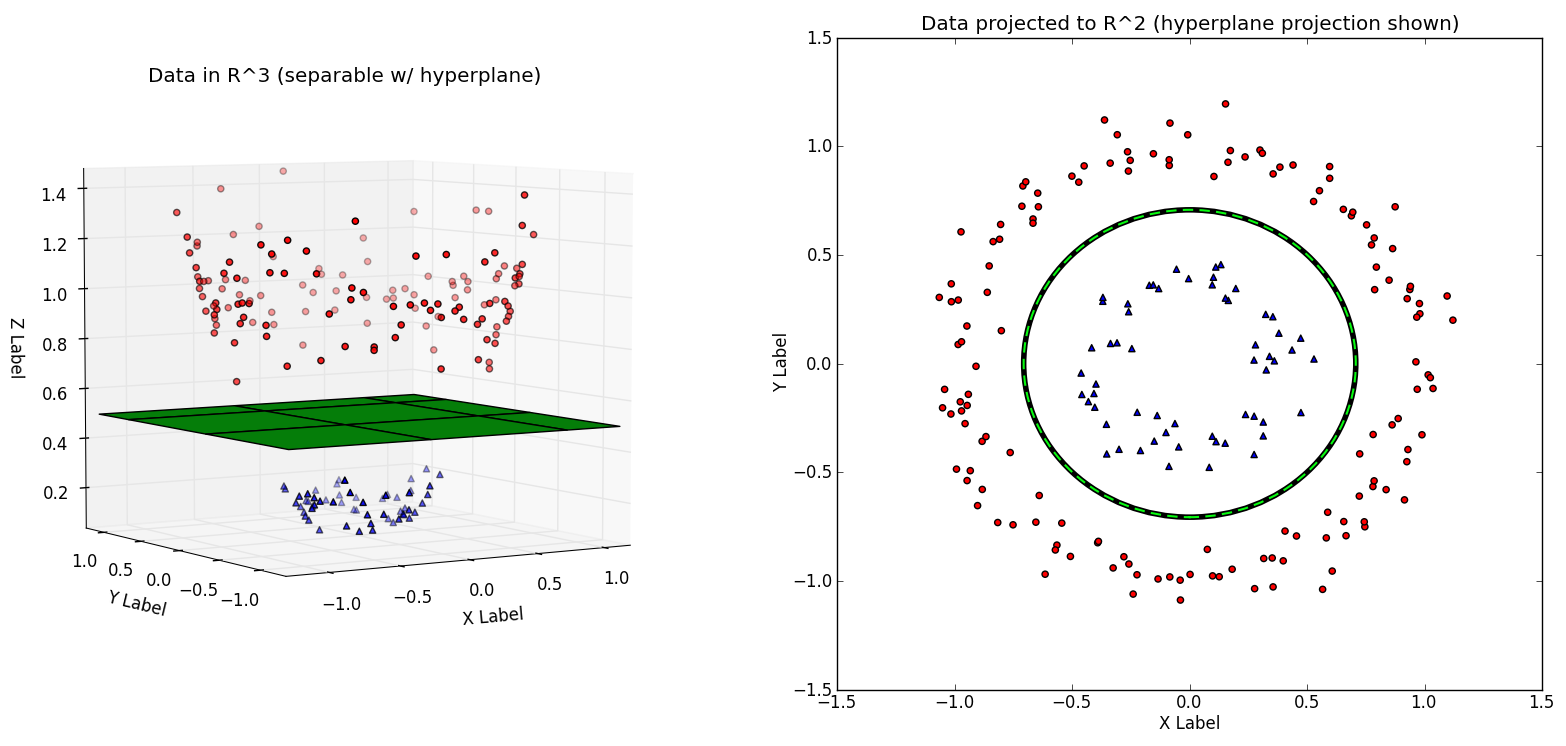Figure 6: (Left) The decision boundary $\vec{w}$ shown to be linear in $\mathbb{R}^3$. (Right) The decision boundary $\vec{w}$, when transformed back to $\mathbb{R}^2$, is nonlinear.

### Recap

A dataset $D$ that is not linearly separable in $\mathbb{R}^N$ may be linearly separable in a higher-dimensional space $\mathbb{R}^M$ (where M > N). Thus, if we have a transformation $\phi$ that lifts the dataset $D$ to a higher-dimensional $D'$ such that $D'$ is linearly separable, then we can train a linear SVM on $D'$ to find a decision boundary $\vec{w}$ that separates the classes in $D'$. Projecting the decision boundary $\vec{w}$ found in $\mathbb{R}^M$ back to the original space $\mathbb{R}^N$ will yield a nonlinear decision boundary.

This means that we can learn nonlinear SVMs while still using the original Linear SVM formulation!

Here are two fantastic animated visualizations of this concept:

The above recap is the key concept that motivates "kernel" methods in machine learning. If you stopped reading here, you will still have the main idea why we use kernels in machine learning tasks. However, there is more to the story (plus, we haven't gotten to kernels yet!).

### Caveat: Impractical for large dimensions

The scheme described so far is attractive due to its simplicity: we only modify the inputs to a 'vanilla' linear SVM. However, consider the computational consequences of increasing the dimensionality from $\mathbb{R}^N$ to $\mathbb{R}^M$ (with $M > N$). If $M$ grows very quickly with respect to $N$ (e.g. $M \in O(2^N)$), then learning SVMs via dataset transformations will incur serious computational and memory problems!

Here is a concrete example: the Polynomial Kernel is a kernel often used with SVMs. For a dataset in $\mathbb{R}^2$, a two-degree polynomial kernel (implicitly) performs the transformation $[x_1, x_2] = [{x_1}^2, {x_2}^2, \sqrt{2} \cdot x_1 \cdot x_2, \sqrt{2 \cdot c} \cdot x_1, \sqrt{2 \cdot c} \cdot x_2, c]$. This transformation adds three additional dimensions $\mathbb{R}^2$ -> $\mathbb{R}^5$.

In general, a d-dimensional polynomial kernel maps from $\mathbb{R}^N$ to an ${N+d \choose d}$-dimensional space . Thus, for datasets with large dimensionality, naively performing such a transformation will quickly become intractable.

Are we hosed? Well, as it turns out...

### We only need the dot products!

It turns out that the SVM has no need to explicitly work in the higher-dimensional space at training or testing time. One can show  that during training, the optimization problem only uses the training examples to compute pair-wise dot products $\langle \vec{x_i}, \vec{x_j} \rangle$, where $\vec{x_i}, \vec{x_j} \in \mathbb{R}^N$.

Why is this significant? It turns out that there exist functions that, given two vectors v and w in $\mathbb{R}^N$, implicitly computes the dot product between v and w in a higher-dimensional $\mathbb{R}^M$ without explicitly transforming v and w to $\mathbb{R}^M$. Such functions are called kernel functions, $K(\vec{v}, \vec{w})$. The implications are:

1. By using a kernel $K(\vec{x_i}, \vec{x_j})$, we can implicitly transform datasets to a higher-dimensional $\mathbb{R}^M$ using no extra memory, and with a minimal effect on computation time.
• The only effect on computation is the extra time required to compute $K(\vec{x_i}, \vec{x_j})$. Depending on $K$, this can be minimal.
2. By virtue of (1), we can efficiently learn nonlinear decision boundaries for SVMs simply by replacing all dot products in the SVM computation with $K(\vec{x_i}, \vec{x_j})$!
The usage of kernel functions to achieve benefits (1) and (2) is the "Trick" in the "Kernel Trick".

### Kernel Functions

In this context, a Kernel function is a function $K: \mathbb{R}^N \times \mathbb{R}^N \rightarrow \mathbb{R}$. There are some important mathematical properties that must be obeyed in order to be considered a proper kernel function: see  (Sec. 3.2-3.3) for a discussion about these properties.

#### Intuition

A kernel $K$ effectively computes dot products in a higher-dimensional space $\mathbb{R}^M$ while remaining in $\mathbb{R}^N$. In symbols:

For $\vec{x_i}, \vec{x_j} \in \mathbb{R}^N, ~K(\vec{x_i},\vec{x_j}) = \langle \phi(\vec{x_i}), \phi(\vec{x_j}) \rangle _M$, where $\langle \cdot, \cdot \rangle _M$ is an inner product of $\mathbb{R}^M$, M > N, and $\phi(\vec{x})$ transforms $\vec{x}$ to $\mathbb{R}^M$ $(\phi: \mathbb{R}^N \rightarrow \mathbb{R}^M)$.

I hope that the reader is able to appreciate this surprising result. At least to me, it seems surprising that we can compute dot products between $\vec{v}, \vec{w} \in \mathbb{R}^N$ in $\mathbb{R}^M$ with a function $K$ that works exclusively in $\mathbb{R}^N$.

### Popular Kernels

Most off-the-shelf classifiers allow the user to specify one of three popular kernels: the polynomial, radial basis function, and sigmoid kernel. For instance, sklearn's SVM implementation svm.SVC has a kernel parameter which can take on linear, poly, rbf, or sigmoid . You can even pass in a custom kernel.

For the following, let $\vec{x_i}, \vec{x_j} \in \mathbb{R}^N$ be rows from the dataset $X$.

1. Polynomial Kernel: $(\gamma \cdot \langle \vec{x_i} , \vec{x_j} \rangle + r)^d$
2. Radial Basis Function (RBF) Kernel: $exp(-\gamma \cdot \lvert \vec{x_i} - \vec{x_j} \rvert ^2)$, where $\gamma > 0$
3. Sigmoid Kernel: $tanh(\langle \vec{x_i}, \vec{x_j} \rangle + r)$
• Author Note: For some reason, sklearn's svm.SVC appears to use both the gamma and coef0 parameters for the kernel='sigmoid', despite the above definition only having one parameter r. I'm not sure what is going on under the hood in sklearn's sigmoid kernel, but not cross-validating across both gamma and coef0 resulted in degenerate decision boundaries (e.g. always assigning -1 to every test example).
Unfortunately, choosing the 'correct' kernel is a nontrivial task, and may depend on the specific task at hand. No matter which kernel you choose, you will need to tune the kernel parameters to get good performance from your classifier. Popular parameter-tuning techniques include K-Fold Cross Validation .

## Back to our Example...

Let's apply the Kernel Trick to the linearly nonseparable dataset in Figure 3. Figures 6-8 show the decision boundaries (along with chosen parameters found via cross validation) for the polynomial, RBF, and sigmoid kernels. As we can see, the resulting SVMs are able to learn high-quality decision boundaries through the application of kernels. For more information about the training processes, such as parameter selection ranges, refer to the sklearn output following the figures: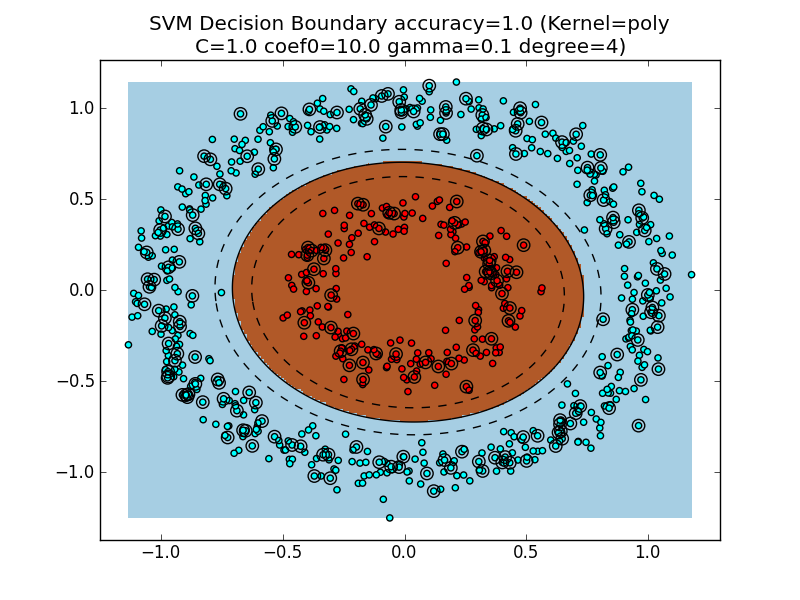Figure 6: The decision boundary with a Polynomial kernel.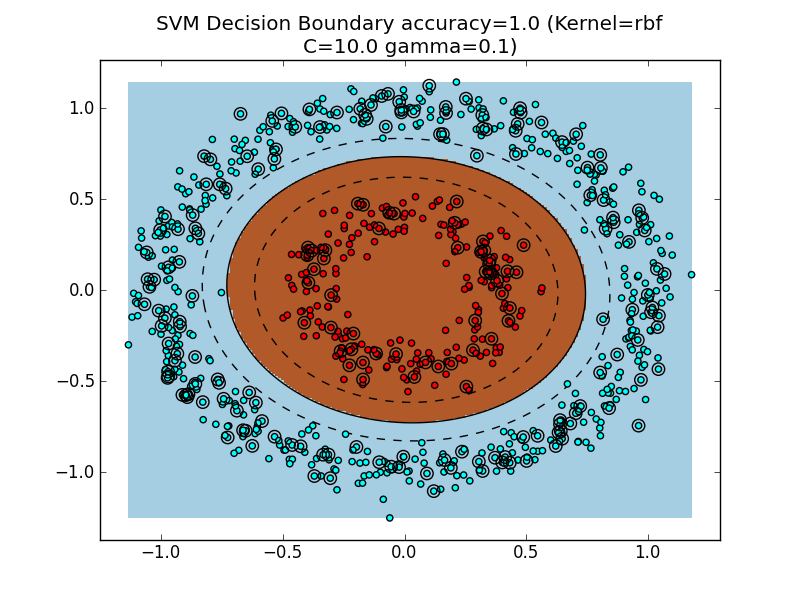Figure 7: The decision boundary with a Radial Basis Function (RBF) kernel.

Author's Note: The Polynomial and RBF kernels found very similar decision boundaries. Interestingly, the Sigmoid kernel resulted in a slightly 'wobbly' decision boundary - this behavior may simply stem from the choice of parameters, rather than from some intrinsic property of the Sigmoid kernel (I'm not sure).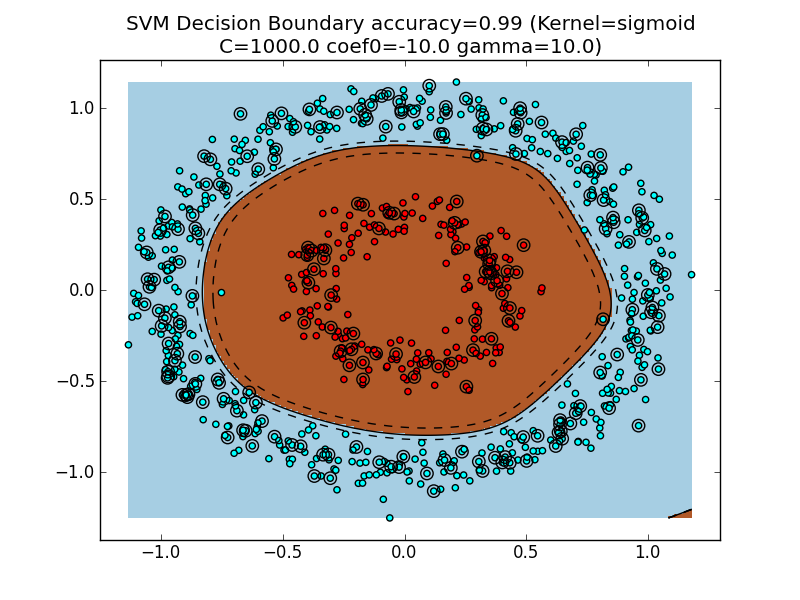Figure 8: The decision boundary with a Sigmoid kernel.

======== Running NONSEPARABLE demo ========
==== Evaluating Random Classifier
== Accuracy: 0.45
precision    recall  f1-score   support

0       0.74      0.42      0.53       151
1       0.23      0.55      0.33        49

avg / total       0.62      0.45      0.48       200

==== Finished Random Classifier (0.000 s)

==== Evaluating SVM (kernel='linear'), 2-fold cross validation
Parameters to be chosen through cross validation:
C: [1.0, 10.0, 100.0, 1000.0, 10000.0]
== Best Params: {'kernel': 'linear', 'C': 1.0}
== Best Score: 0.476666666667
== Accuracy: 0.445
precision    recall  f1-score   support

0       0.78      0.37      0.50       151
1       0.26      0.67      0.37        49

avg / total       0.65      0.45      0.47       200

==== Finished Linear SVM (1.290 s)

==== Evaluating SVM (kernel='poly'), 2-fold cross validation
Parameters to be chosen through cross validation:
C: [1.0, 10.0, 100.0, 1000.0]
coef0: [1.0, 10.0, 100.0]
gamma: [0.001, 0.01, 0.1]
degree: [2, 4]
== Best Params: {'kernel': 'poly', 'C': 1.0, 'coef0': 10.0, 'gamma': 0.1, 'degree': 4}
== Best Score: 1.0
== Accuracy: 1.0
precision    recall  f1-score   support

0       1.00      1.00      1.00       151
1       1.00      1.00      1.00        49

avg / total       1.00      1.00      1.00       200

==== Finished Polynomial SVM (2.290 s)

==== Evaluating SVM (kernel='rbf'), 2-fold cross validation
Parameters to be chosen through cross validation:
C: [1.0, 10.0, 100.0, 1000.0, 10000.0]
gamma: [0.0001, 0.001, 0.01, 0.1]
== Best Params: {'kernel': 'rbf', 'C': 10.0, 'gamma': 0.1}
== Best Score: 1.0
== Accuracy: 1.0
precision    recall  f1-score   support

0       1.00      1.00      1.00       151
1       1.00      1.00      1.00        49

avg / total       1.00      1.00      1.00       200

==== Finished RBF Kernel (0.555 s)

==== Evaluating SVM (kernel='sigmoid'), 2-fold cross validation
Parameters to be chosen through cross validation:
C: [0.1, 1.0, 10.0, 100.0, 1000.0, 100000.0]
coef0: [-10000.0, -1000.0, -100.0, -10.0, 1.0, 10.0, 100.0]
gamma: [0.001, 0.01, 0.1, 1.0, 10.0, 100.0]
== Best Params: {'kernel': 'sigmoid', 'C': 1000.0, 'coef0': -10.0, 'gamma': 10.0}
== Best Score: 0.991666666667
== Accuracy: 0.99
precision    recall  f1-score   support

0       1.00      0.99      0.99       151
1       0.96      1.00      0.98        49

avg / total       0.99      0.99      0.99       200

==== Finished Sigmoid SVM (5.995 s)

...Finished. Total Time: 10.330 s


## Summary

We review the main points of this writeup:

1. Brief review of Linear SVMs for Binary Classification.
2. Encountered a linearly non-separable dataset that a Linear SVM is not able to handle.
3. Data which is linearly nonseparable in $R^N$ may be linearly separable in a higher-dimensional space $\mathbb{R}^M$ ( $M > N$, where $N$ is the original feature space dimensionality).
4. Unfortunately, a naive implementation of (3) would result in a massive time and space burden. For instance, for the $d$-degree polynomial kernel, the new feature space dimensionality $M$ grows at a rate of ${N + d \choose d}$.
5. However, the SVM formulation only requires dot products $\langle \vec{x_i}, \vec{x_j} \rangle$ between training examples $\vec{x_i}, \vec{x_j} \in X$ (where $X$ is the training data).
6. By replacing all dot products $\langle \vec{x_i}, \vec{x_j} \rangle$ with a kernel function $K(\vec{x_i}, \vec{x_j})$, we can implicitly work in a higher-dimensional space $\mathbb{R}^M$ ( $M > N$), without explicitly building the higher-dimensional representation. Thus, the SVM can learn a nonlinear decision boundary in the original $\mathbb{R}^N$, which corresponds to a linear decision boundary in $\mathbb{R}^M$.
• This is the "trick" in "Kernel trick"
• Machine Learning folks say that we have increased the "expressiveness" of Linear SVMs. Recall that the vanilla Linear SVM can only learn linear decision boundaries in $\mathbb{R}^N$. By introducing kernel methods, Linear SVMs can now learn nonlinear decision boundaries in $\mathbb{R}^N$.
• Remember: the decision boundary will still be linear in $\mathbb{R}^M$, the feature space induced by the kernel $K$!
7. Finally, we empirically evaluated SVMs with various kernels, and observed a significant improvement when the dataset is not linearly separable.

## Closing Remarks

I hope that this writeup has provided an approachable explanation of the "Kernel Trick". I make a deliberate effort to emphasize the high-level concepts that demonstrate the intuition behind kernel methods. If the reader is interested in a more mathematically rigorous handling of kernel methods, I direct the reader to several fantastic reference material that I referred to while creating this article.

 was prepared by Professor Michael Jordan (University of California, Berkeley) for a Spring 2004 offering of CS 281-B (Statistical Learning Theory). The article covers the same points that this writeup touches upon, but precisely shows how we can replace dot products $\langle \vec{x_i}, \vec{x_j} \rangle$ with kernel functions $K(\vec{x_i}, \vec{x_j})$ within the SVM mathematical formulation.  is a set of lecture slides prepared by Professor Robert Berwick (MIT), and provides a detailed overview of the SVM in an easy-to-understand format.

## Future Work

An illuminating exercise would be to investigate the effects of poor parameter choices for kernel methods. Because using a kernel adds additional parameters to the model (for instance, the RBF kernel has the $\gamma$ parameter), proper model selection is critical to achieve good performance.

Also, an interesting question to explore is: for a given dataset $D$, does there always exist a kernel $K$ such that a linear SVM perfectly separates the class labels with a hyperplane?

Finally, it would be neat to take real-world datasets and compare the performance of various kernels on various classification tasks. Synthetic datasets offer a convenient way to illustrate concepts, but actually seeing a nontrivial example is pretty exciting.

## Acknowledgements

Several interactive components of this page are enabled by the Twitter Bootstrap (http://twitter.github.com/bootstrap/) and JQuery plugins (http://jquery.com/). The inline LaTeX equations are provided by CodeCogs (http://www.codecogs.com/index.php). They request that the following image be displayed: .

## Source Code

Here are links to the code used to generate the figures and empirical results:

## Release Notes

• 1-9-2013
• Initial release.
• 12-20-2017
• Added PDF version of article, in response to reports that equations were not being rendered on the web page.